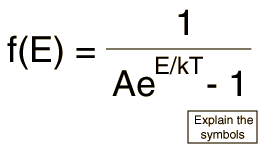The Bose-Einstein Distribution

The Bose-Einstein distribution describes the statistical behavior of integer spin particles (bosons). At low temperatures, bosons can behave very differently than fermions because an unlimited number of them can collect into the same energy state, a phenomenon called "condensation".Distribution Functions Numerical example
Index

Applied statistics concepts

 HyperPhysics***** Quantum Physics R Nave
Go Back

Bose-Einstein DetailsThe Bose-Einstein distribution.
Index

Applied statistics concepts

 HyperPhysics***** Quantum Physics R Nave
Go Back